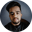Related Tags

c++
oop
inheritance
communitycreator

# How to call a base class function from a derived class functionShubham Singh Kshatriya

### Overview

In this shot, we'll learn how to call a base class function from a derived class function in C++.

### Syntax

We can call a base class function from a derived class using the following syntax:

baseClassName :: functionName();
Syntax

Now, let's see some examples.

### The base class

Let's first create a base class.

class base {
public:
void display () {
cout << "Function of base class" << endl;
}
}
Base class

### Explanation

• Line 1: We define a class base.
• Lines 3–5 : We create a function display().

### The derived class

Let's create a derived class by extending the base class.

class derived: public base {
public:
void display () {
cout << "Function of derived class" << endl;

// calling base class function
base :: display();
}
}
Derived class

### Explanation

• Line 1: We define a class derived by extending the class base.
• Line 3–8: We override the base class function display().
• Line 7: We call the base class function display() from the derived class.

### Example

#include <bits/stdc++.h>
using namespace std;

class base {
public:
void display () {
cout << "Function of base class" << endl;
}
};

class derived: public base {
public:
void display () {
cout << "Function of derived class" << endl;

// calling base class function
base :: display();
}
};

int main() {
// create object of derived class
derived d;
d.display();
return 0;
}
Calling a base class function from the derived class

### Explanation

• Line 1: We import the bits/stdc++.h library.
• Line 4–19: We create a base class and a derived class.
• Line 21–26: Inside the main() function, we create an object of the derived class and call its display() function.
• On calling the display() function of the derived class:
• It prints "Function of derived class" on the console.
• It calls the display() function of the base class.
• It prints "Function of base class" on the console.

RELATED TAGS

c++
oop
inheritance
communitycreator

CONTRIBUTORShubham Singh Kshatriya
RELATED COURSES

View all Courses

Keep Exploring

Learn in-demand tech skills in half the time